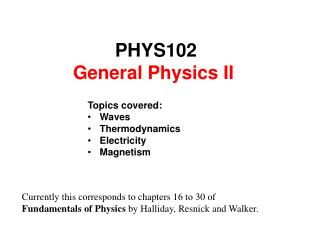Download PresentationPHYS102 General Physics II

# PHYS102 General Physics II  - PowerPoint PPT PresentationDownload Presentation## PHYS102 General Physics II

- - - - - - - - - - - - - - - - - - - - - - - - - - - E N D - - - - - - - - - - - - - - - - - - - - - - - - - - -
##### Presentation Transcript

1. PHYS102 General Physics II  • Topics covered: • Waves • Thermodynamics • Electricity • Magnetism Currently this corresponds to chapters 16 to 30 of  Fundamentals of Physics by Halliday, Resnick and Walker.

2. Syllabus and teaching strategy Lecturer: GolibjonBerdiyorov Room 148, Building 6 Phone: 860-3869/2283 e-mail: golib@kfupm.edu.sa Office Hours: Sunday-Thursday: 11.00-12.00 Lectures: Sunday: 9.00 – 9.50 (6/125) 07-09 Tuesday: 9.00 – 9.50 (6/125) 07-09 Thursday: 9.00 – 9.50 (6/125) 07-09 Recitation: Wednesday: 7.00 – 7.50 (6/106) 07 9.00 – 9.50 (6/103) 08 10.00 – 10.50 (6/106) 09

4. Chapter 16 Waves

5. Waves and particles

6. Types of Waves

7. Transverse and longitudinal waves Motion of particles and wave

8. Transverse and longitudinal waves Motion of particles and wave Neither transverse or longitudinal waves

9. Transverse and longitudinal waves Motion of particles and wave

10. Wavelength and frequency Sinusoidal wave

11. Wavelength and frequency Sinusoidal wave

12. Wavelength and frequency Sinusoidal wave

13. Wavelength and frequency Sinusoidal wave

14. Wavelength and frequency Sinusoidal wave

15. Wavelength and frequency Sinusoidal wave

16. Wavelength and frequency Two ways to put the wave on paper

17. Wavelength and frequency Two ways to put the wave on paper

18. Wavelength and frequency Wavelength and period

19. Wavelength and frequency Mathematical representation of a wave

20. Wavelength and frequency Mathematical representation of a wave

21. Wavelength and frequency Mathematical representation of a wave

22. Wavelength and frequency Mathematical representation of a wave

23. Wavelength and frequency Mathematical representation of a wave

24. Wavelength and frequency Mathematical representation of a wave

25. Wavelength and frequency Mathematical representation of a wave

26. Wavelength and frequency Mathematical representation of a wave

27. Wavelength and frequency Mathematical representation of a wave

28. Wavelength and frequency Changing the position by one wavelength

29. Wavelength and frequency Changing time by one period

30. Wavelength and frequency Angular wave number and angular frequency

31. Wavelength and frequency Angular wave number displacement distance

32. Wavelength and frequency Angular frequency displacement time

33. Phase constant

34. Wavelength and frequency Checkpoint

35. Wavelength and frequency Checkpoint

36. Wavelength and frequency Checkpoint

37. Wavelength and frequency Checkpoint

38. 16.2.1. According to the text, waves are of three main types. Which of the following choices correctly lists these three main types? a) mechanical, sound, and light waves b) mechanical, electromagnetic, and matter waves c) transverse, sound, and matter waves d) longitudinal, electromagnetic, and sound waves e) simple harmonic, light, and matter waves

39. 16.2.1. According to the text, waves are of three main types. Which of the following choices correctly lists these three main types? a) mechanical, sound, and light waves b) mechanical, electromagnetic, and matter waves c) transverse, sound, and matter waves d) longitudinal, electromagnetic, and sound waves e) simple harmonic, light, and matter waves

40. 16.2.2. What type of waves are composed of electrons, protons, and other fundamental particles? a) electromagnetic b) longitudinal c) nuclear resonant d) matter e) nanotrophic

41. 16.2.2. What type of waves are composed of electrons, protons, and other fundamental particles? a) electromagnetic b) longitudinal c) nuclear resonant d) matter e) nanotrophic

42. 16.3.1. Which one of the following types of waves is purely longitudinal? a) light traveling through vacuum b) waves on a plucked guitar string c) radio waves traveling through air d) sound waves emitted from a speaker e) surface waves on the surface of a shallow pond

43. 16.3.1. Which one of the following types of waves is purely longitudinal? a) light traveling through vacuum b) waves on a plucked guitar string c) radio waves traveling through air d) sound waves emitted from a speaker e) surface waves on the surface of a shallow pond

44. 16.3.2. Which one of the following statements concerning transverse waves is true? a) The direction of the disturbance is parallel to the direction of travel. b) The direction of the disturbance is perpendicular to the direction of travel. c) A sound wave is an example of a transverse wave. d) Transverse waves are not periodic waves. e) Transverse waves always travel at the speed of light.

45. 16.3.2. Which one of the following statements concerning transverse waves is true? a) The direction of the disturbance is parallel to the direction of travel. b) The direction of the disturbance is perpendicular to the direction of travel. c) A sound wave is an example of a transverse wave. d) Transverse waves are not periodic waves. e) Transverse waves always travel at the speed of light.

46. 16.3.3. A sound wave is an example of what type of wave? a) longitudinal wave b) electromagnetic wave c) matter wave d) transverse wave e) seismic wave

47. 16.3.3. A sound wave is an example of what type of wave? a) longitudinal wave b) electromagnetic wave c) matter wave d) transverse wave e) seismic wave

48. 16.4.1. Which one of the following expressions determines the angular wave number? a) b) c) d) e)

49. 16.4.1. Which one of the following expressions determines the angular wave number? a) b) c) d) e)